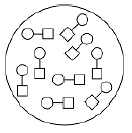# Problem: Let a small circle represent an atom of one type of element and a small square represent an atom of a second type of element. Select what is shown on each of the pictures:(a) a pure substance (a compound) composed of the two elements (in a one-to-one ratio)(b) a homogeneous mixture composed of the two elements(c) a heterogeneous mixture composed of the two elements

###### FREE Expert Solution

The given is:83% (401 ratings)###### Problem Details

Let a small circle represent an atom of one type of element and a small square represent an atom of a second type of element. Select what is shown on each of the pictures:(a) a pure substance (a compound) composed of the two elements (in a one-to-one ratio)
(b) a homogeneous mixture composed of the two elements
(c) a heterogeneous mixture composed of the two elements

Frequently Asked Questions

What scientific concept do you need to know in order to solve this problem?

Our tutors have indicated that to solve this problem you will need to apply the Classification of Matter concept. You can view video lessons to learn Classification of Matter. Or if you need more Classification of Matter practice, you can also practice Classification of Matter practice problems.